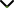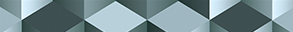# Advances in Fractional Functional Analysis

Publishing date
01 Feb 2022
Status
Closed
01 Oct 2021

Guest Editors

1São Paulo State University (UNESP), São José do Rio Preto, Brazil

2Baba Farid College, Bathinda, India

3University of Catania, Catania, Italy

This issue is now closed for submissions.

# Advances in Fractional Functional Analysis

This issue is now closed for submissions.

## DescriptionIn the last decade, fractional calculus became popular and important due its application in several research fields such as mathematics, physics, engineering, etc. Nowadays, fractional calculus is widely applied in electromagnetism, dynamical systems, partial differential equations (PDE), etc. Fractional calculus represents one of the most interesting research fields in contemporary mathematics.

Several fractional operators have found many real-world applications due to their properties of interpolation between operators of integer order. In addition, fractional function spaces have been widely applied for solving differential integral, integro-differential equations both in pure and applied mathematics. In the last twenty years, fractal operators have become of interest to researchers. Several publications have discussed these fractal operators and have talked about the connection between fractal operators and the wavelet analysis. Consequently, fractional functional analysis plays the role of the link between wavelet analysis, fractional geometry, and more in general, between different fields of applied functional analysis. In particular, the fractional functional analysis extends the concept of function spaces to function spaces of fractional dimensions, opening up new frontiers both in functional analysis and fractional calculus.

The aim of this Special Issue is to bring together original research and review articles discussing recent advances in fractional calculus from a more general point of view. Theoretical and practical studies in pure and applied mathematics are welcome.

Potential topics include but are not limited to the following:

• Fractional differential equations
• Fractional function spaces
• Commutators of fractional integral operators
• Fractional calculus via Mittag-Leffler functions
• Leibniz algebras, fractional calculus, and function spaces of symmetric functions
• Fractional differential and integral equations
• Fractional calculus, function space and approximation theory
• Fractional models in applied sciencesJournal metrics
See full report
Acceptance rate23%
Submission to final decision72 days
Acceptance to publication20 days
CiteScore2.600
Journal Citation Indicator1.430
Impact Factor1.9Author guidelinesEditorial boardDatabases and indexing

Article of the Year Award: Impactful research contributions of 2022, as selected by our Chief Editors. Discover the winning articles.